# How to calculate fractions with different denominators

Want to find out how to calculate fractions with different denominators? We've provided a step-by-step guide of how to calculate fractions with different denominators to teach you everything you need to know.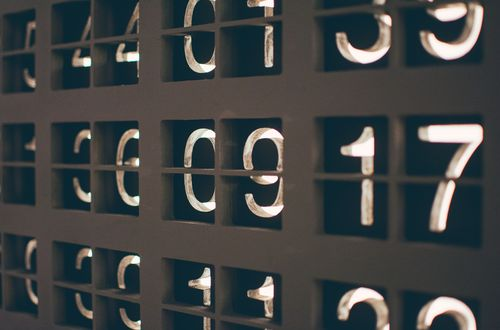## Step 1: Determine the least common denominator

If the fractions have different denominators, determine the least common denominator (LCD) shared by the fractions.

## Step 2: Convert to the same denominator

Express the fractions so that they share the same denominator using the LCD.

## Step 3: Add or subtract the numerators

Once the denominator of the fractions are the same, add or subtract the given numerators.

## Step 4: Simplify the fractions

Cancel out common factors and simplify the fractions if needed.

## Examples of calculating fractions with different denominators

Q1) Perform the indicated operations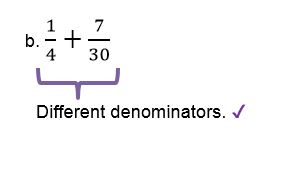Since the denominators are different, let’s first look for the LCD.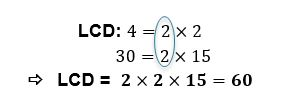Express the fractions with the LCD as their denominator.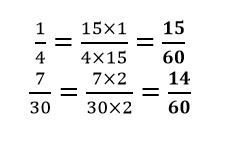Once the denominators are the same, we can subtract the numerators to find the difference.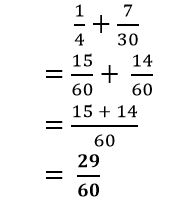#### Join today

The fastest way to practice

Unlock our complete testing platform and improve faster that ever.